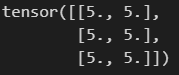# Pytorch register full backward pre hook can not change the grad of the module

The simplest code is follows:

``````import torch
from torch import nn

l1 = nn.Linear(2,3)
l1.register_full_backward_pre_hook(hook)

x = torch.rand(5,2)
y = l1(x)
loss = y.sum()
loss.backward()

``````

pytorch version is 2.0.1+cu118. Is there a bug? If I dont annotate the `x.requires_grad_(True)`, it would be right.

From the docs:

The hook should not modify its arguments, but it can optionally return a new gradient with respect to the output that will be used in place of grad_output in subsequent computations.

I think the output of `l1.weight.grad` in this code should be a large tensor since I have added a big number into the grad of `l1` layer’s output at the backward_pre stage.

However, when I run the code:

``````import torch
from torch import nn

return a

l1 = nn.Linear(2,3)
l1.register_full_backward_pre_hook(hook)

x = torch.ones(5,2)
y = l1(x)
loss = y.sum()
loss.backward()

``````

It output:When I run:

``````import torch
from torch import nn

return a

l1 = nn.Linear(2,3)
l1.register_full_backward_pre_hook(hook)

x = torch.ones(5,2)The only difference between the two codes is in `x.requires_grad_(True)`. I think that the results of two runs should both be tensors of 50000000, or, at least the results of both should be the same.